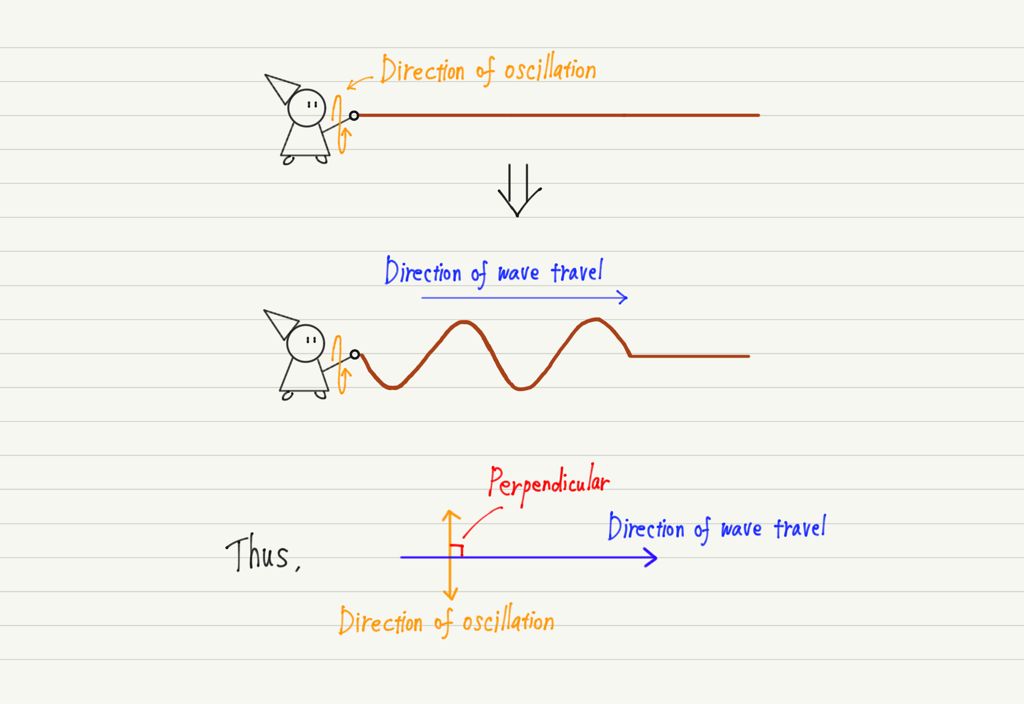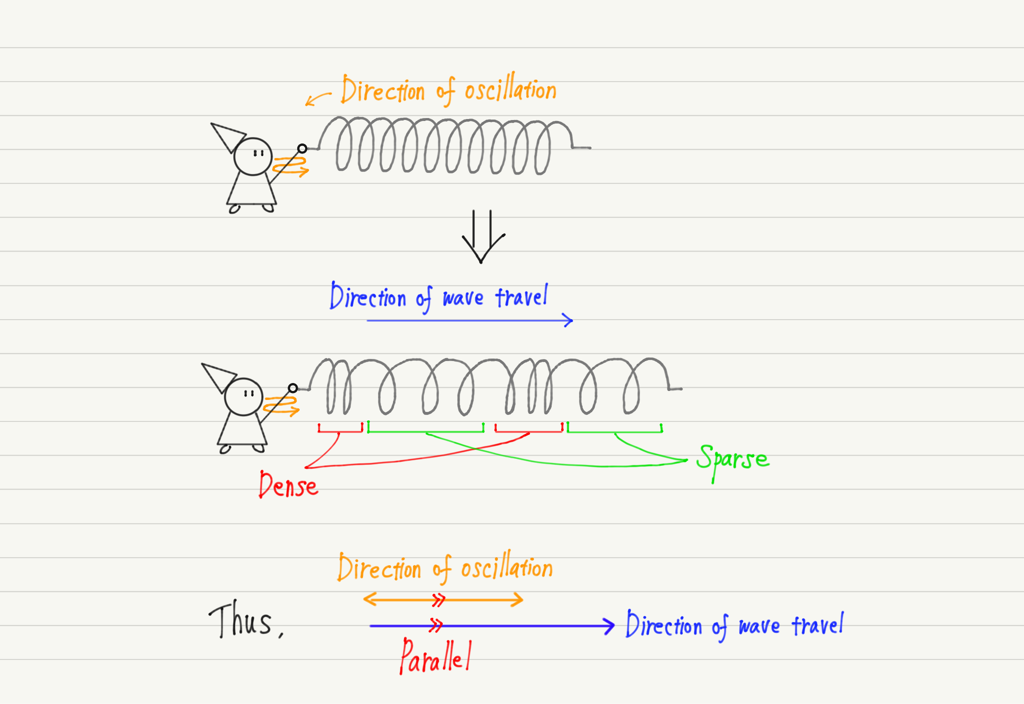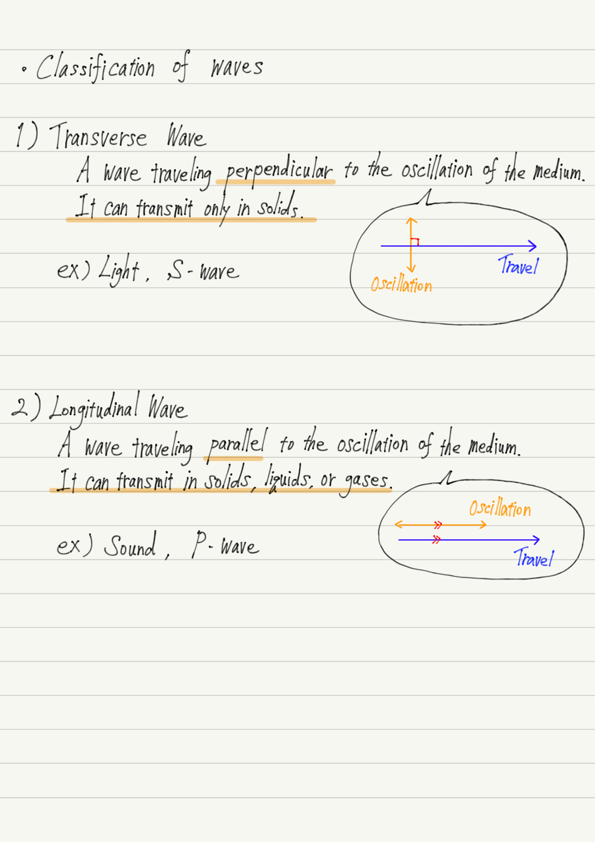# Transverse Wave and Longitudinal Wave

A wave is a phenomenon in which oscillations are transmitted to the surroundings.
We have used the example of a rope to visualize oscillations, but there are waves that propagate in a different way than waves propagating through a rope.

In this lecture, you will learn the classification of waves according to the way vibrations are transmitted.
There are two types of classification, which are called transverse wave and longitudinal wave.

## Transverse wave

The wave that travels along the rope we have seen so far is a typical example of a transverse wave.

A transverse wave is a wave in which the direction of oscillation of the medium and the direction of wave travel are perpendicular.

If you are not familiar with this, imagine a rope. If you hold the end of the rope and swing it up and down, the waves will move away from you.
A simple diagram of this is shown below.Indeed, it can be seen that the direction of oscillation and the direction of travel are perpendicular to each other for waves traveling along a rope.

What properties do transverse waves have?
Continue to imagine a rope. Then, cut the rope in the middle.

No wonder, no oscillation is transmitted beyond the point where it is cut!

In fact, the same is true in the atomic world.
For transverse waves to propagate, atoms must be attached to each other, and if atoms are in a disjointed state, such as in a liquid or gas, transverse waves cannot propagate to the surrounding area.

Therefore, transverse waves can only propagate in solids.

Examples of non-rope transverse waves include light and S-wave of earthquake.

## Longitudinal wave

Next is the longitudinal wave.
A longitudinal wave is a wave in which the direction of oscillation of the medium and the direction of wave travel are parallel.

Unlike transverse waves, for which there is an easy-to-understand example of a rope, longitudinal waves are difficult to visualize.
The image of a longitudinal wave is the oscillation of a spring.Now, let’s prepare a spring and create a longitudinal wave.
Hold the spring and oscillate it in the direction of expansion and contraction.When a spring is vibrated as shown in the above figure, the contracted part tries to extend and the extended part tries to contract due to elastic force.
This is a longitudinal wave that moves forward while repeating this process.

Transverse waves move forward with alternating crests and troughs, whereas longitudinal waves move forward with alternating “shrinking (dense)” and “stretching (sparse)” areas.

The essence of longitudinal wave propagation is its tendency to return to its original state.
I used a spring as an example because it is the easiest to understand, but all substances have the property of trying to return to their original state.
Water and air are no exception.

Therefore, longitudinal waves can propagate through all solids, liquids, and gases.

Incidentally, sound is the most familiar and important example of a longitudinal wave.
Other examples of longitudinal wave is the P-wave of earthquake.

## Summary of this lectureWe have studied transverse and longitudinal waves, but not all waves belong to one or the other.
It is known that waves, such as those traveling on the surface of water, are neither transverse nor longitudinal.

## Next Time

Let’s consider how to represent a longitudinal wave on a graph.

https://www.yukimura-physics.com/entry/wave-f09

error: Content is protected !!